|

# Rehome&YVVY助欢乐颂诠释陪伴背后家的味道

5月11日《欢乐颂2》强势回归，一经播出就稳坐收视率第一的位置。深挖《欢乐颂2》能够续写霸屏的原因，除了剧中反映的社会现实引人深思外，五美本季中更时尚且具有个性的同款也为电视剧吸引了更多拥趸。家，是心境与个性的体现

随着《欢乐颂2》的热播，几个姐妹也迎来了不同的新生活：安迪与小包总甜腻的普吉岛之旅，樊姐将要面临的新追求者，曲筱绡到了要“见家长”的阶段，小蚯蚓终于不被渣男虐遇见了呆萌的程序员应勤，而一向安静的“乖乖女”关雎尔本季感情也将会有大突破。

除了剧中主演们复杂的感情线外，我们也能从五美的家居风格中看出一些剧组刻画出的人物个性来。

安迪的家低调，相比颜值来讲吸引人的更多是极富质感的细节，这也反映出她考究的品位和独立自信的人格；樊胜美的家充满了众多像抱枕这样的品质物件，因为这能让樊姐找到心理上的安定，提示她过的还不错；曲筱绡的家中每个大胆混搭的时尚设计，都带着她的肆意洒脱和无所畏惧；“傻白甜”的小蚯蚓邱莹莹，再困难的事一个卡通小抱枕就能复苏她快乐的心，这也反映出了她乐观积极的性格；而对于“乖乖女”关雎尔来说，一本书，一杯茶，一个抱枕于她，既是文艺的气场，更是满怀暖心的陪伴。

家，是心境与个性的体现，电视剧也在通过风格迥异的软装，给观众们一些关于主演性格的暗示，以及提供了解五美心境的环境。

曲筱绡+YVVY 肆意洒脱与前卫时尚的碰撞

本季中曲筱绡与赵医生的恋爱再次升级，这对平时打打闹闹撒狗粮的CP在新危机中感情也在面临着种种考验。刚结束的18集中赵医生要带曲筱绡见家长，但身为大学老师的招妈妈和工程师的赵爸爸，让一向洒脱的曲筱绡紧张起来，抱着黑色猫咪抱枕大喊“怎么办“。这一场景中YVVY的黑色猫咪抱枕让曲筱绡的俏皮可爱体现得淋漓尽致。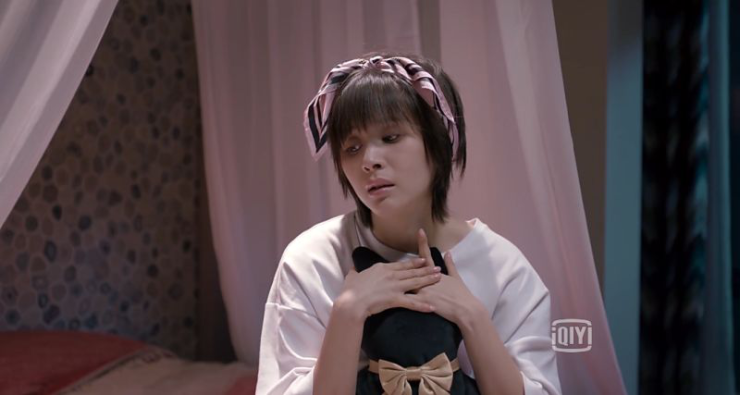（曲筱绡抱着YVVY黑色猫咪抱枕，苦恼如何优雅地见赵医生爸妈）

曲筱绡的家居大部分都是YVVY的家居产品，大到沙发的时尚设计，小到一个猫咪抱枕的俏皮可爱，透过YVVY的家居风格，我们也能立体地看到曲筱绡古灵精怪时尚洒脱的个性特点。

YVVY在剧中不仅仅是一件件家具，更是生活中潮酷符号的代表。YVVY是美克美家旗下品牌，专为个性独具而生，和所有的不可能一拍即合，以酷、潮、惊喜、独特的品牌价值主张引领前沿时尚风格，并通过多元工艺与元素的跨界混搭，将个性态度极致彰显，让电视前的观众在追剧的同时遇见久违的个性共鸣。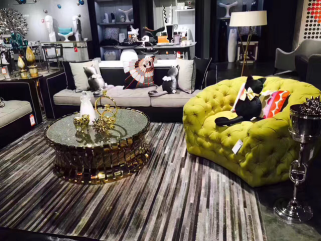（剧中曲筱绡前卫时尚又俏皮可爱的家居由YVVY提供）

Rehome助阵 带你重新定义五美的家

第一季中五个姑娘的着装被“找同款”从头扒到尾，在第二季里五美的家也被360度无死角剖析，樊姐的抱枕、小蚯蚓的香薰还有曲筱绡的猫咪抱枕等同款产品刷爆了朋友圈。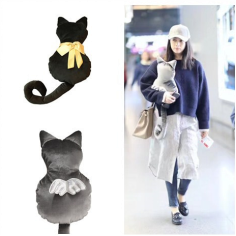（蒋欣拍戏时超级喜欢的银色猫咪，是直接把它抱回家了吗？）

第三集中，安迪从普吉岛回来为大家送上礼物，送给小蚯蚓的精美蜡烛香薰，就是来自Rehome家居的Rigaud香薰。Rigaud产于法国，是世界上最早的香水制作商之一，成分天然、纯度极高等特点让奥黛丽•赫本、肯尼迪夫人都曾是它的忠实粉丝，香气虽淡，却能传递出强烈的情感；再说第八集中，关关与小蚯蚓为庆祝樊胜美换了新工作，特意送给她一个Rehome的抱枕，这也让樊胜美不安的心中有了一些温暖。礼物背后是情感的关怀与爱意的表达，而这些看似没有生命的物件，却能像22楼的姐妹们一样，有了它的陪伴，好像再艰难的生活也能苦中带着点甜。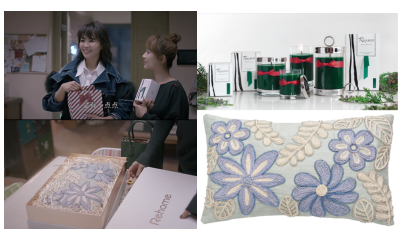（Rigaud法国里高香氛蜡烛、Rehome抱枕）

作为《欢乐颂2》的指定家居合作品牌，Rehome将会通过家居饰品的衬托让人物的性格和喜好展现得更淋漓尽致，也会继续为剧情的发展增添光彩。而Rehome的大量家居产品除了会在剧中抢眼出境，剧外更有“五美”定制卡通人物形象的联名款抱枕推出！5月11日起，Rehome APP独家全面预售五美同框款抱枕，活动期间下载注册APP即可得100元现金券，APP内无门槛直接消费；更有满额送活动，APP内消费满499，即可赠送五美抱枕一个。

活动详情请莅临Rehome全国门店或下载“用馨送”APP了解活动公告，也可关注Rehome官方微信提前了解更多《欢乐颂2》幕后的精彩故事。（《欢乐颂2》睡衣趴发布会 Rehome五美定制抱枕抢眼）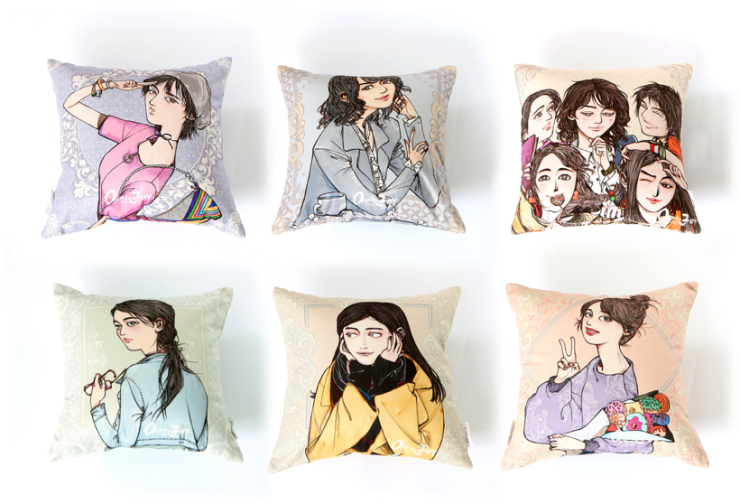（Rehome《欢乐颂2》五美定制抱枕单品图）

Rehome是美克美家旗下子品牌。品牌秉承“温馨、雅致、愉悦、便捷”的价值主张，为热爱生活、富有创造力、品味高雅的消费者甄选全球创意家居用品，并设计开发家具产品，满足当下不断变换的家居需求。温馨，是家的温度，是爱的味道。您对温馨生活的渴望，我们感同身受。

`声明：本文由入驻焦点开放平台的作者撰写，除焦点官方账号外，观点仅代表作者本人，不代表焦点立场错误信息举报电话： 400-099-0099，邮箱：jubao@vip.sohu.com，或点此进行意见反馈，或点此进行举报投诉。`A B C D E F G H J K L M N P Q R S T W X Y Z
A - B - C - D - E
• A
• 鞍山
• 安庆
• 安阳
• 安顺
• 安康
• 澳门
• B
• 北京
• 保定
• 包头
• 巴彦淖尔
• 本溪
• 蚌埠
• 亳州
• 滨州
• 北海
• 百色
• 巴中
• 毕节
• 保山
• 宝鸡
• 白银
• 巴州
• C
• 承德
• 沧州
• 长治
• 赤峰
• 朝阳
• 长春
• 常州
• 滁州
• 池州
• 长沙
• 常德
• 郴州
• 潮州
• 崇左
• 重庆
• 成都
• 楚雄
• 昌都
• 慈溪
• 常熟
• D
• 大同
• 大连
• 丹东
• 大庆
• 东营
• 德州
• 东莞
• 德阳
• 达州
• 大理
• 德宏
• 定西
• 儋州
• 东平
• E
• 鄂尔多斯
• 鄂州
• 恩施
F - G - H - I - J
• F
• 抚顺
• 阜新
• 阜阳
• 福州
• 抚州
• 佛山
• 防城港
• G
• 赣州
• 广州
• 桂林
• 贵港
• 广元
• 广安
• 贵阳
• 固原
• H
• 邯郸
• 衡水
• 呼和浩特
• 呼伦贝尔
• 葫芦岛
• 哈尔滨
• 黑河
• 淮安
• 杭州
• 湖州
• 合肥
• 淮南
• 淮北
• 黄山
• 菏泽
• 鹤壁
• 黄石
• 黄冈
• 衡阳
• 怀化
• 惠州
• 河源
• 贺州
• 河池
• 海口
• 红河
• 汉中
• 海东
• 怀来
• I
• J
• 晋中
• 锦州
• 吉林
• 鸡西
• 佳木斯
• 嘉兴
• 金华
• 景德镇
• 九江
• 吉安
• 济南
• 济宁
• 焦作
• 荆门
• 荆州
• 江门
• 揭阳
• 金昌
• 酒泉
• 嘉峪关
K - L - M - N - P
• K
• 开封
• 昆明
• 昆山
• L
• 廊坊
• 临汾
• 辽阳
• 连云港
• 丽水
• 六安
• 龙岩
• 莱芜
• 临沂
• 聊城
• 洛阳
• 漯河
• 娄底
• 柳州
• 来宾
• 泸州
• 乐山
• 六盘水
• 丽江
• 临沧
• 拉萨
• 林芝
• 兰州
• 陇南
• M
• 牡丹江
• 马鞍山
• 茂名
• 梅州
• 绵阳
• 眉山
• N
• 南京
• 南通
• 宁波
• 南平
• 宁德
• 南昌
• 南阳
• 南宁
• 内江
• 南充
• P
• 盘锦
• 莆田
• 平顶山
• 濮阳
• 攀枝花
• 普洱
• 平凉
Q - R - S - T - W
• Q
• 秦皇岛
• 齐齐哈尔
• 衢州
• 泉州
• 青岛
• 清远
• 钦州
• 黔南
• 曲靖
• 庆阳
• R
• 日照
• 日喀则
• S
• 石家庄
• 沈阳
• 双鸭山
• 绥化
• 上海
• 苏州
• 宿迁
• 绍兴
• 宿州
• 三明
• 上饶
• 三门峡
• 商丘
• 十堰
• 随州
• 邵阳
• 韶关
• 深圳
• 汕头
• 汕尾
• 三亚
• 三沙
• 遂宁
• 山南
• 商洛
• 石嘴山
• T
• 天津
• 唐山
• 太原
• 通辽
• 铁岭
• 泰州
• 台州
• 铜陵
• 泰安
• 铜仁
• 铜川
• 天水
• 天门
• W
• 乌海
• 乌兰察布
• 无锡
• 温州
• 芜湖
• 潍坊
• 威海
• 武汉
• 梧州
• 渭南
• 武威
• 吴忠
• 乌鲁木齐
X - Y - Z
• X
• 邢台
• 徐州
• 宣城
• 厦门
• 新乡
• 许昌
• 信阳
• 襄阳
• 孝感
• 咸宁
• 湘潭
• 湘西
• 西双版纳
• 西安
• 咸阳
• 西宁
• 仙桃
• 西昌
• Y
• 运城
• 营口
• 盐城
• 扬州
• 鹰潭
• 宜春
• 烟台
• 宜昌
• 岳阳
• 益阳
• 永州
• 阳江
• 云浮
• 玉林
• 宜宾
• 雅安
• 玉溪
• 延安
• 榆林
• 银川
• Z
• 张家口
• 镇江
• 舟山
• 漳州
• 淄博
• 枣庄
• 郑州
• 周口
• 驻马店
• 株洲
• 张家界
• 珠海
• 湛江
• 肇庆
• 中山
• 自贡
• 资阳
• 遵义
• 昭通
• 张掖
• 中卫

1室1厅1厨1卫1阳台

1
2
3
4
5

0
1
2

1

1

0
1
2
3报名成功，资料已提交审核A B C D E F G H J K L M N P Q R S T W X Y Z
A - B - C - D - E
• A
• 鞍山
• 安庆
• 安阳
• 安顺
• 安康
• 澳门
• B
• 北京
• 保定
• 包头
• 巴彦淖尔
• 本溪
• 蚌埠
• 亳州
• 滨州
• 北海
• 百色
• 巴中
• 毕节
• 保山
• 宝鸡
• 白银
• 巴州
• C
• 承德
• 沧州
• 长治
• 赤峰
• 朝阳
• 长春
• 常州
• 滁州
• 池州
• 长沙
• 常德
• 郴州
• 潮州
• 崇左
• 重庆
• 成都
• 楚雄
• 昌都
• 慈溪
• 常熟
• D
• 大同
• 大连
• 丹东
• 大庆
• 东营
• 德州
• 东莞
• 德阳
• 达州
• 大理
• 德宏
• 定西
• 儋州
• 东平
• E
• 鄂尔多斯
• 鄂州
• 恩施
F - G - H - I - J
• F
• 抚顺
• 阜新
• 阜阳
• 福州
• 抚州
• 佛山
• 防城港
• G
• 赣州
• 广州
• 桂林
• 贵港
• 广元
• 广安
• 贵阳
• 固原
• H
• 邯郸
• 衡水
• 呼和浩特
• 呼伦贝尔
• 葫芦岛
• 哈尔滨
• 黑河
• 淮安
• 杭州
• 湖州
• 合肥
• 淮南
• 淮北
• 黄山
• 菏泽
• 鹤壁
• 黄石
• 黄冈
• 衡阳
• 怀化
• 惠州
• 河源
• 贺州
• 河池
• 海口
• 红河
• 汉中
• 海东
• 怀来
• I
• J
• 晋中
• 锦州
• 吉林
• 鸡西
• 佳木斯
• 嘉兴
• 金华
• 景德镇
• 九江
• 吉安
• 济南
• 济宁
• 焦作
• 荆门
• 荆州
• 江门
• 揭阳
• 金昌
• 酒泉
• 嘉峪关
K - L - M - N - P
• K
• 开封
• 昆明
• 昆山
• L
• 廊坊
• 临汾
• 辽阳
• 连云港
• 丽水
• 六安
• 龙岩
• 莱芜
• 临沂
• 聊城
• 洛阳
• 漯河
• 娄底
• 柳州
• 来宾
• 泸州
• 乐山
• 六盘水
• 丽江
• 临沧
• 拉萨
• 林芝
• 兰州
• 陇南
• M
• 牡丹江
• 马鞍山
• 茂名
• 梅州
• 绵阳
• 眉山
• N
• 南京
• 南通
• 宁波
• 南平
• 宁德
• 南昌
• 南阳
• 南宁
• 内江
• 南充
• P
• 盘锦
• 莆田
• 平顶山
• 濮阳
• 攀枝花
• 普洱
• 平凉
Q - R - S - T - W
• Q
• 秦皇岛
• 齐齐哈尔
• 衢州
• 泉州
• 青岛
• 清远
• 钦州
• 黔南
• 曲靖
• 庆阳
• R
• 日照
• 日喀则
• S
• 石家庄
• 沈阳
• 双鸭山
• 绥化
• 上海
• 苏州
• 宿迁
• 绍兴
• 宿州
• 三明
• 上饶
• 三门峡
• 商丘
• 十堰
• 随州
• 邵阳
• 韶关
• 深圳
• 汕头
• 汕尾
• 三亚
• 三沙
• 遂宁
• 山南
• 商洛
• 石嘴山
• T
• 天津
• 唐山
• 太原
• 通辽
• 铁岭
• 泰州
• 台州
• 铜陵
• 泰安
• 铜仁
• 铜川
• 天水
• 天门
• W
• 乌海
• 乌兰察布
• 无锡
• 温州
• 芜湖
• 潍坊
• 威海
• 武汉
• 梧州
• 渭南
• 武威
• 吴忠
• 乌鲁木齐
X - Y - Z
• X
• 邢台
• 徐州
• 宣城
• 厦门
• 新乡
• 许昌
• 信阳
• 襄阳
• 孝感
• 咸宁
• 湘潭
• 湘西
• 西双版纳
• 西安
• 咸阳
• 西宁
• 仙桃
• 西昌
• Y
• 运城
• 营口
• 盐城
• 扬州
• 鹰潭
• 宜春
• 烟台
• 宜昌
• 岳阳
• 益阳
• 永州
• 阳江
• 云浮
• 玉林
• 宜宾
• 雅安
• 玉溪
• 延安
• 榆林
• 银川
• Z
• 张家口
• 镇江
• 舟山
• 漳州
• 淄博
• 枣庄
• 郑州
• 周口
• 驻马店
• 株洲
• 张家界
• 珠海
• 湛江
• 肇庆
• 中山
• 自贡
• 资阳
• 遵义
• 昭通
• 张掖
• 中卫• 手机• 分享
• 设计
免费设计
• 计算器
装修计算器
• 入驻
合作入驻
• 联系
联系我们
• 置顶
返回顶部